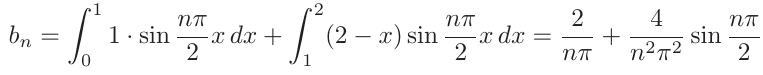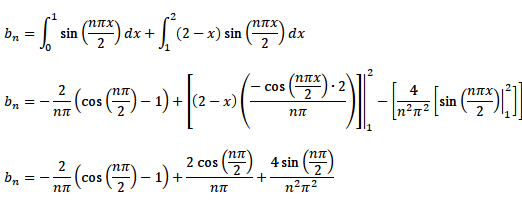# Definite integral

## Homework Statement## The Attempt at a SolutionCould you write some sentences too? You just posted a bunch of equations. So, could you tell us exactly the statement that needs to be proven. Tell us in words what you did. Tell us exactly where you are stuck.

Could you write some sentences too? You just posted a bunch of equations. So, could you tell us exactly the statement that needs to be proven. Tell us in words what you did. Tell us exactly where you are stuck.

bn needs to be computed. The solution is under 1.

I computed bn and the end result is under 3. The issue is that my end result doesn't match the solution's end result.

Office_Shredder
Staff Emeritus
Gold Member
You got the same answer, just cancel the positive and negative
$$\frac{2}{n\pi} \cos\left( \frac{n\pi}{2} \right)$$
that you have

You got the same answer, just cancel the positive and negative
$$\frac{2}{n\pi} \cos\left( \frac{n\pi}{2} \right)$$
that you have

Thanks. These fourier series problems are so painfully long T_T.